﻿ Braking of the space crafts caused by the vacuum fluctuations. Effect Pioneer anomaly. Cosmological redshift. Hubble constant. De Broglie waves. Big Bang theory. Casimir effect.
 Sergey B. Alemanov  http://alemanow.narod.ru  alemanow@mail.ru Braking of the space crafts causedby the vacuum fluctuations

## One of the consequences of the dynamical Casimir effect.

«The reason of the existence of the Casimir effect are the energetical fluctuations of the vacuum that occur as a result of permanent appearance and disappearance in it of the virtual particles.»
http://ru.wikipedia.org/wiki/Эффект_Казимира (In Russian)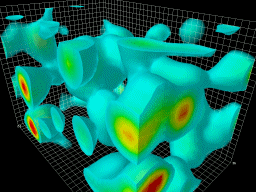Vacuum fluctuations.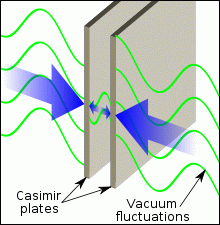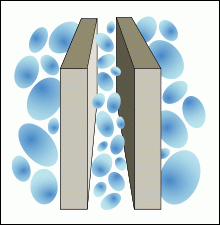The pressure of the vacuum fluctuations on the parallel plates. The static Casimir effect.

In 2011 physicists have registered for the first time the dynamical Casimir effect, it has been recognized as the most important discovery of the year. They managed to transfer the energy of the vacuum fluctuations into the real particles (e.g., photons), with other words, they managed to extract the energy out of the vacuum.

«Allocating the major discoveries of 2011, the 'Nature' magazine has put on the first place the discovery of the dynamical Casimir effect.»
http://elementy.ru/lib/431579 (In Russian)
«In the late May last year (2011), many of the popular newspapers had following headlines: "Scientists have received energy out the vacuum!"»
http://www.popmech.ru/article/10440-energiya-vakuuma (In Russian)
«According to the quantum theory, the vacuum is not just a void. It has constant energy fluctuations: the birth and the death of the pairs of the virtual particles and antiparticles. In spite of its virtuality, they can exert pressure. This is called The static Casimir effect and it has already been confirmed experimentally. In theory, there is also The dynamical Casimir effect - the conversion of the vacuum fluctuations into the real particles (e.g., photons). This effect was for the first time observed by the scientists.»
http://zoom.cnews.ru/rnd/news/top/effekt_kazimira_na_praktike_svet_rodilsya_iz_nichego (In Russian)

If the vacuum fluctuations, introducing virtual particles, are able to put pressure on the plates, then the moving plates, respectively, can also "push" the virtual particles - dynamical Casimir effect. It should be noted that the STR does not prohibit the movement relative to the virtual particles, as well as, for example, relative to the microwave background radiation, that is while moving, the plates are allowed to "push" the virtual particles.

«A system of rapidly rotating mirrors, converting virtual photons into the real ones, allowed for the first time to demonstrate the dynamical Casimir effect.»
http://www.popmech.ru/article/9145-dinamicheskaya-razvirtualizatsiya/ (In Russian)
«... emerging from the vacuum pairs of the virtual particles at the sufficiently high speed can, thanks to energy they receive from the mirror, be separated and converted into the real particles.»
http://ufn.ru/ru/news/2011/7/ (In Russian)
«In dynamical Casimir effect vacuum fluctuations are being converted into the real photons. The kinetic energy of the mirror is thus being spent on the birth of the photons.»
http://elementy.ru/lib/431579 (in Russian)

The transfer of the kinetic energy to the virtual particles - is, with other words, the inhibition of the mirrors against the vacuum fluctuations. Dynamical Casimir effect presents direct evidence of the fact that braking of the bodies in the vacuum does exist. The higher the speed, the greater is the loss of the kinetic energy per time unit.

«... mirror transfers some of its kinetic energy to virtual photons, which helps them to materialise.»
http://www.chalmers.se/en/news/Pages/Chalmers-scientists-create-light-from-vacuum.aspx

The movement of the mirrors with respect to the fluctuations of the physical vacuum converts virtual photons into the real ones (it is clear that the energy of the appearing photons does not exceed the loss of the kinetic energy of the mirrors). Thus it is experimentally confirmed, that a moving body can "push" virtual particles. Consequently it leads to the dissipation (loss) of the kinetic energy which is being converted into the energy of the fluctuations, transforming the virtual particles into the real ones. With other words, the dynamical Casimir effect represents the friction against the vacuum fluctuations (the friction against the virtual particles). Experimental confirmation of the dynamical Casimir effect once again proves the correctness of the quantum physics in the statement that the vacuum is "the sea" of the virtual particles.

«Dynamical Casimir effect is based on a similar idea - a conducting uncharged plate, moving in the space with a sufficiently high speed (ideally - with the speed comparable to the speed of light), prevents the disappearance of the virtual photons "pushing" some of them forward. As a result, by the viewer such a plate will be seen as one emitting photons.»
http://nayki.ru/news156061.html (In Russian)
«At such speeds the main contribution to the friction will be made by the quantum fluctuations.»
The "Nature" magazine. 2011. 9. P.13. (In Russian)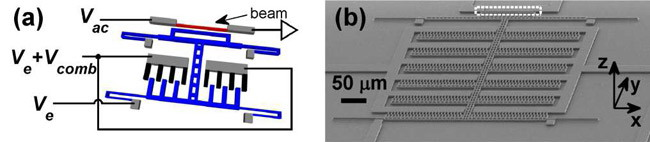«The engineers have created a silicon chip capable of measuring the Casimir effect - the pressure of virtual particles on the close located plates.»
http://lenta.ru/news/2012/07/31/kasimirchip/ (In Russian)

A compact device (microchip), which measures the pressure of the vacuum, was created. Now it is possible to measure and compare the pressure in different areas such as the surface of the Earth or space. Not so long ago the static Casimir effect - vacuum pressure - seemed unbelievable. Now the dynamical Casimir effect - friction in the vacuum - received its experimental confirmation. Once again, the laws of quantum physics break the usual stereotypes.

«Experiment confirming the dynamical Casimir effect was first proposed at the LPI RAS.»
http://www.fian-inform.ru/?mode=mnews&id=1076&page=1 (In Russian)
«In 2011 a group of scientists from the Chalmers' University of Technology confirmed the dynamical Casimir effect.»
http://ru.wikipedia.org/wiki/Эффект_Казимира (In Russian)
«Chalmers scientists create light from vacuum»
http://www.chalmers.se/en/news/Pages/Chalmers-scientists-create-light-from-vacuum.aspx
«Physicists from Sweden, USA and Japan have described the first observation of the dynamical Casimir effect.»
http://www.modcos.com/news.php?id=97 (In Russian)

Quantum vacuum fluctuations are not only able to exert pressure and push the bodies to get closer to each other (static Casimir effect), but also can they brake the speed of the spacecrafts due to the fact that a moving body "pushes" fluctuations forward (dynamical Casimir effect). Since all particles (bodies) in quantum physics are represented in the form of the the de Broglie waves, it is obvious that the calculations should be made also on the basis of the quantum-wave representations. The formula for the dissipation (the loss) of the kinetic energy for the period of one fluctuation of the de Broglie wavelength is deduced: WT = H0hc/v ("viscosity of the physical vacuum" formula), where H0 - Hubble constant, h - Planck constant, c - velocity of light, v - particle (body) speed. This formula permits direct calculations of the cosmological redshift and the space crafts braking ("Pioneer" space crafts anomaly).

Unlike researches, where the influence of the vacuum fluctuations on the resting bodies was studied (static Casimir effect), this work analyzes the influence of the vacuum fluctuations on the moving bodies, where besides the pressure of the fluctuations one also observes the friction (the braking of the bodies) as one of the consequences of the dynamical Casimir effect. It is clear that if there is pressure, the resistance to the movement should certainly also occur. The existence of the vacuum fluctuations interacting which the matter means that the vacuum is not an ideal environment for the movement. At first this article describes the dissipation of the photon energy, and then, following the principle of the corpuscular-wave dualism, a generalization applicable to all elementary particles and physical bodies is made.

«At present it is ascertained that vacuum is not an "empty space". It is certain material continuum with quite determined, but unclear properties. It was confirmed by vacuum effects observation such as "zero-oscillations", vacuum polarization, particle generation by electromagnetic interactions. Therefore it is reasonable to suggest that physical vacuum could have real own internal "friction" resistance due to its own small but real viscosity. Thus, photons could interact with vacuum which could result in astronomical redshift of the light.»
http://bourabai.narod.ru/shtyrkov/evolution.htm (in Russian).
«In real matter expanding of waves is always followed by the energy loss (dissipation) as a result of energy transformation into heat.»
Russian Encyclopedia on Physics. Chapter "Waves" (in Russian).

If vacuum field fluctuations exist, then in this vacuum there also would be electromagnetic wave energy absorption - a wave's energy transforms into fluctuation energy. It is clear that if the vacuum fluctuations can be converted into real photons, the reverse process should also be possible. If in the formula of the cosmological redshift we would replace the frequency by the photon's energy z = (E0 - Ez)/Ez , then it becomes evident, that at the same redshift higher the photon's energy (frequency) is, the higher is the energy loss zEz = (E0 - Ez). That is to say that the energy loss (dissipation) is directly proportional to the elapsed oscillation number! It is not difficult to calculate the amount of the photon's energy loss per one oscillation: ET = EZT = EH0T = hvH0T = hH0 = 1.6·10-51 J, where E - is photon energy E = hv, h - Planck constant, v - photon's frequency, ZT - redshift per one oscillation ZT = H0T, H0 - Hubble constant (2.4 ± 0.12)·10-18 s-1(Hz), T - oscillation period T = 1/v. The amount by which the photon frequency decreases for one period of oscillation: vZT = vH0T = H0 = 2.4·10-18 Hz. For example, when photon 6·1014 Hz (E = 3.98·10-19 J), have passed 40 megaparsecs distance - r = 1.234·1024 m, - it has done r/λ = rv/c = 2.47·1030 amount of oscillations, where λ - wave length λ = c/v, c - velocity of light. Respectively, its energy loss (dissipation) is 2.47·1030 × 1.6·10-51 J = 3. 95·10-21 J, and redshift is - z = 3.95·10-21 J / 3.98·10-19 J = 0.01. That is to say that irrespectively from the photon's frequency with every photon's wave oscillation it loses part of the energy ET = hH0, which is being transformed into the vacuum fluctuation energy. The Hubble constant is only the value, that decreases the photon frequency during one period of oscillation, regardless of wavelength. Such frequency change is totally consistent with the observed cosmological redshift, and the link between the period of oscillation and the Hubble constant directly points to the fact that there is dissipation of wave's energy. In every environment the distribution of the waves is always accompanied by the loss (dissipation) of the energy due to the transformation of this energy into the inner energy of this environment (fluctuation). Distribution of the waves in physical vacuum is also not an exception, as - according to the quantum physics - a vacuum is not an emptiness. In it just as in any other environment fluctuations named "zero-oscillations" take place.

«The term "physical vacuum" has appeared. It means "community" of the virtual particles emerging for the short periods of time and then disappearing again. According to the modern knowledge, they appear in pares "particle-anti-particle" and then disappear as the result of the annihilation. Thus, virtual couples "electron-positron" annihilate with generation of the virtual photon, which transforms itself into "electron-positron" pare, and so on... Appearance and disappearance of the virtual particles is "quantum fluctuations". Because any fluctuations are changes around some average number, the physical vacuum could be described as quantum system in minimal energy state, with its energy averaging to zero. Therefore quantum fluctuations of the vacuum are often called "zero-oscillations" of the electromagnetic field. ... In addition, they may have effects on the real particles and fields existing in the vacuum.»
"American physicists generated something from nothing." http://www.nkj.ru/archive/articles/5158/ (In Russian)

That is to say that the real and the virtual particles are constantly interacting with each other and the transformation of the vacuum fluctuations into the real particles and vice versa can take place. For example, fluctuations of the vacuum (zero-point fluctuations of the electromagnetic field) can be converted into real photons - microwave background radiation (the spectrum of the "black body" with a temperature of 2.7 K), and the virtual electrons take the role of real and vice versa - the Lamb shift.

«And now, let's imagine: we observe this flying particle (let it be an electron), and next to it virtual pares appear and disappear. Often Nature confuses virtual particles with real ones, because one electron is exactly the same as the other one. So imagine that close to your observed electron a virtual pare appeared, only the antiparticle confused its virtual particle with the real one and annihilated with the real particle. As a result of that, the virtual electron has no other choice as to take upon a role of the real particle. As result we see a true "miracle" - particle disappears in one place and suddenly appears in another. A true teleportation indeed. Such "shaking" of the electron orbit in atom was theoretically predicted and experimentally confirmed - "lamb shift".»
"Casimir effect or vacuum problem." http://www.eduhmao.ru/var/db/files/8630.kasimir.doc (In Russian)

The virtual particles are absolutely same as the real particles only they live very short time, that is why they are called virtual. It was experimentally confirmed that these virtual particles in spite of their short lifetime influence the movement of the real particles (lamb shift). That is to say that physical vacuum being a matter, influences with its fluctuations the movement of all particles and, respectively, influences the movement of any physical objects.

«Quantum field theory declares that according to the "uncertainty principle" in physical vacuum virtual particles constantly appear and disappear. With other words - "zero-oscillations" of fields constantly take place.»
http://ru.wikipedia.org/wiki/Вакуум (In Russian)

The movement between the virtual particles to certain extent is similar to the movement in the real matter, because the physical vacuum filled with virtual particles is a material environment.

«Virtual particles determine the properties of the physical vacuum which acquires in modern physics the properties of the material environment.»
http://ru.wikipedia.org/wiki/Материя_(физика) (In Russian)

Virtual particles present invisible (dark) matter, filling the vacuum, therefore when photon moves in vacuum it still interacts with virtual particles, resulting in energy loss. That is to say that the photon moves not in an "empty space", but in the "field environment", consisting of the "sea" of the virtual particles. Charged virtual particles being under the influence of the changing electromagnetic field begin its own oscillations just like real particles do. It should be stressed that virtual particles can not diffract, because in order to be able to diffract a particle should at first absorb and then eject photon. This is however forbidden for the virtual particles because they exist in an unlinked state (no atoms). The pure field interaction takes place, because electrical and magnetic currents in electromagnetic wave influence the charged virtual particles by shifting them (vacuum polarization). At the same time the energy of the wave is being partially spent causing redshift. Such interaction is not being dependent upon the wave length, because resonance does not happen. Another example of pure photon fields interaction is when photons loose their energy (become red) in gravitation field, where photons also do not diffract. The fact that electromagnetic wave fades, expanding in field environment, is not an extraordinary fact as literally any material environment causes wave fading. Light consists of photons and the smaller is the energy of the photon, the larger is the length of its wave.

It is impossible to divide macro- and micro-world, therefore we should remember that all particles and bodies are de Broglie waves with their own length and frequency. Sometimes de Broglie waves are being interpreted as probability waves, but "probability" is pure mathematical term and has no relation to diffraction and interference. There is no need in such idealistic interpretations now - when it is commonly accepted that vacuum is just one of the types of the matter, represented by quantum fields with least energy level. Interference is redistribution of the vibrational energy in the environment, that is why only real waves are capable of the generating of the diffraction and the interference, and this statement is also applicable to de Broglie waves. Waves without energy do not exist as any wave is an expanding oscillation and it causes energy transformation from one form into another and back. In such physical process the loss of the energy (dissipation) always takes place. The energy is being transformed into the inner energy of the environment. De Broglie waves (kinetic energy waves) just as any other waves lose their energy with time - or transform it into the inner energy of the vacuum (energy of the vacuum fluctuation). This is seen as ""Pioneer" space craft anomaly effect".

«One of important results of "Pioneer" and "Voyager" space craft missions is space craft braking effect, ""Pioneer" anomaly effect". Nature of this effect remains unknown.»
http://www.cnews.ru (in Russian)
«Immediately after the discovery of the anomaly in the Jet Propulsion Laboratory of NASA an expert group led by John Anderson was created to discover the source of the anomaly. Taking to account the fact that such anomaly effect is not being influenced by time factor or craft position, the scientists have considered the influence of all possible sources of such constant non-gravity-related factors: gas leaking, space dust collisions, non-equilibrium warmth distribution in the crafts themselves, radio-waves transmitter impulses. However, even after taking to account all of these factors, the anomaly did not disappear. More than that, it turned out that investigations of the space crafts such as "Galileo", "Cassini", "Ulysses" working later outside of the Solar System, revealed same braking effect. This allows to suggest that the reason of such an anomaly is not any of the technical aspects relating to certain space craft. At this moment members of the scientific group believe that the reason of the effect has explanation in the so-called "new-physics".»
http://www.ntsomz.ru/news/news_cosmos/pioneer_29_june_2005 (in Russian)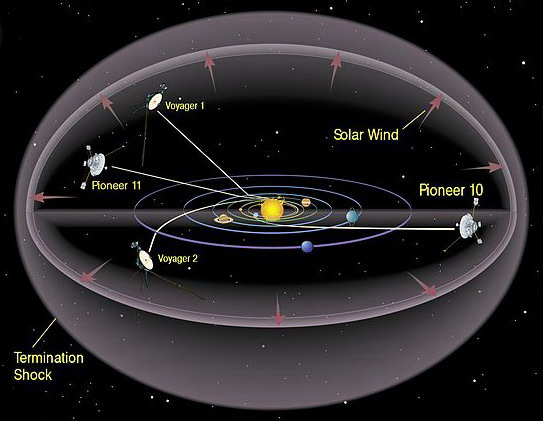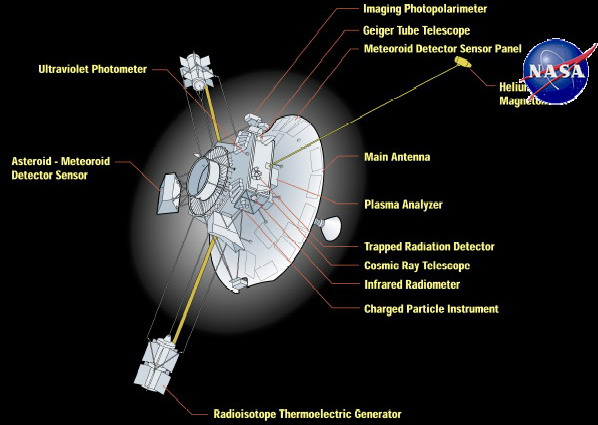It has been discovered that technical aspects are not the reasons of the anomaly. For instance, specialists from Anderson's group engaged in calculations of the heat effect, declared that "the effect of the heat emission in "Pioneer" anomaly braking should be small". They stated that heat effect decreases due to accumulators exhausting, but "Pioneer" braking remains constant. Moreover, different types of the space crafts have same braking value. In an attempt to explain the anomaly by thermal effects certain "fitting" of the facts can be seen: some scientists have one, some other results of calculations, but in any case these effects are small and insufficient for explanation of the anomaly. More precisely the anomalous deceleration is described by formula of the energy's dissipation of one period of de Broglie wave oscillation, which allows to calculate cosmological redshift as well as "Pioneer" space craft anomaly. The formula is universal and applicable to all bodies and particles, including photons: WT = H0hc/v, where c - velocity of light, v - particle (body) speed. For example, if particle (body) 1 g weight (m = 0.001 kg) at the speed of 10000 m/s for 100 years (t = 3155760000 s), then its de Broglie wave has done r/λ = vt/λ = tmv2/h = 4.76·1047, amount of oscillations, and respectively, kinetic energy dissipation (loss) would become WD = (tmv2/h) · (H0hc/v) = H0cvtm = H0cpt = H0crm = 22.7 J, where r - traveled way r = vt, p - impulse p = mv, λ - wave length λ = h/mv. Meanwhile the speed of the particle would decrease to 9997.7 m/s, and redshift of de Broglie wave would become z = (10000 m/s - 9997.7 m/s) / 9997.7 m/s = 0.00023. This formula shows that kinetic energy dissipation is directly proportional to the mass of the particle and the traveled distance - WD = H0crm, as well as to the impulse and the time - WD = H0cpt, that is to say that the higher is the impulse, the more is the loss of the energy per time unit. For example, body with mass 1 kg while travelling 1 m distance loses kinetic energy WD = H0crm = 7.2·10-10 J. Respectively, the resistance force equals to FD = H0cm = 7.2·10-10 N, and the braking value equals to ap = cH0 = (7.2 ± 0.36)·10-10 m/s2. Same braking value ap = (8.74 ± 1.33)·10-10 m/s2 (within the limits of the possible error, plus a small contribution of the thermal radiation) has been received experimentally as a result of the investigation of the "Pioneer" anomaly effect. This proves that WT = H0hc/v formula is correct, its results correspond with the experimental results. If the braking is ap = cH0, then the body moves with 1 m/s speed and it will stop in t = v/0 = 44 years, having traveled r = v2/20 = 700000 km distance, decrease in the velocity - 0.02 m/s per year. The photons can be calculated similarly, but it should be remembered that the energy loss does not result in speed changing. For example, photon energy loss is ED = H0cvtm = H0tE = zE, where E - the photon energy, and per one photon oscillation the energy loss equals to ET = H0hc/v = H0h = 1.6·10-51 J.

Kinetic energy does not exist without de Broglie waves, that is why they are linked to any moving particle, that is to say that kinetic energy represents by itself the de Broglie wave. De Broglie waves are the material essence of the kinetic energy. Real de Broglie waves (not the probability waves) have - just as all physical waves - frequency equal to v = v/λ.

If difficulties arise with the calculation of the speed, it is sufficient to just convert this formula, replacing speed with a wave of de Broglie: WT = H0hc/v = H0λmc (λ = h/mv). This way the dissipation of the kinetic energy during one period of oscillations of the de Broglie wave equals to the length of the de Broglie wave multiplied by the Hubble constant, the mass and the velocity of the light. That is the question of the relativity of movement falls off as it is enough to know the length of de Broglie wave. This formula can be considered precise because it calculates only one period of the wave oscillation. The fact of the small amount of the base constants in the formula shows its fundamentality. This formula is amazing in its simplicity WT = H0hc/v - is true revolution in understanding of the real properties of physical vacuum. The myth about existence in the vacuum of the ideal waves distributing without dissipation is dispelled. The physical vacuum has a field nature where even in its base condition quantum fluctuations (also called "zero-field-oscillations") always take place. All particles - not just photons - are disturbed conditions of the field, representing the wave while moving. Therefore for all particles these waves could be calculated the same way λ = h/p. Respectively, the formula of the dissipation is the same as well - WT = H0hc/v. This formula reflects the fact that apart of such qualities as length, frequency and energy every type of the wave also has its dissipation caused by transformation of one sort of energy into another and back by every fluctuation. Such, though very small, loss of the kinetic energy being transformed into the internal energy of the vacuum (energy of the vacuum fluctuations) provides significant braking for all space objects. The above stated formula helps to make such calculation more precise.

«Scientists established that so-called "Pioneers anomaly" - anomalous braking of space crafts Pioneer 10 and Pioneer 11 in the outer part of the Solar System - has non-gravitational nature.»
http://www.lenta.ru/news/2009/12/21/pioner/ (in Russian)

Anomalous braking was discovered for all space crafts, for which such calculations were technically possible. Moreover, there are facts confirming the braking of the asteroids as well.

«Assumable, the "Pioneers effect" should affect the asteroid considerably. This asteroid is in an completely different location than that one resulting from the astronomers calculations.»
http://science.compulenta.ru/182831/ (in Russian)
«... NASA expert group under the leading of S.G. Turyshev believe that the "Pioneers anomaly" deserves the most detailed attention. It is possible that investigation of this effect may lead to the fundamental changes in the laws of the modern physics.»
http://news.cosmoport.com/2004/11/29/1.htm (in Russian)
«ap = (8.74 ± 1.33)·10-10 m/s2  ...  ap = cH»
http://lnfm1.sai.msu.ru/grav/russian/life/chteniya/sagi2007/turyshev_Anomaly.pdf

NASA investigation group under the leading of S.G. Turyshev came up with a formula ap = cH0, where ap - is acceleration of the anomalous braking (8.74 ± 1.33)·10-10 m/s2, H0 - Hubble constant (2.4 ± 0.12)·10-18 s-1, c - light speed. Although tis formula is given without any extra explanations, it logically leads to the conclusion that the cosmological redshift and the "pioneers anomaly" are two names of the one effect: the effect of the loss of the kinetic energy which is being transformed into the vacuum fluctuation energy. It can be proved by simple calculations. Hubble constant per 1 megaparsec is (74.2 ± 3.6) km/s. Light travels through one megaparsec (3.09·1019 km) in 3.26 million years (1.03·1014 s). When we multiply anomalous braking by this time figure we will receive value that is almost equal to Hubble constant on one megaparsec: (8.74 ± 1.33)·10-10 m/s2 × 1.03·1014 s = (90 ± 13.7) km/s.

It proves that all particles (and bodies) including photons experience anomalous braking. But as photon is wave which always moves with the light speed, they lose only energy, not a speed. Similar situation is seen when the photons lose their energy in the gravitational field, showing redshift, but other particles (bodies) brake, losing speed. It is clear that if the anomalous braking effects the elementary particles, it would also effect the photons as they are particles, too. This leads to the fact that cosmological redshift can be calculated with the help of the anomalous braking constant. Anomalous braking is V = apt, where t - elapsed time. Respectively, de Broglie waves redshift is z = apt/v, where v - particle's velocity. Because all particles obey the principle of the corpuscular-wave dualism, then with this formula we calculate redshift for photons as well: z = apt/c, where c - the speed of the photon (light). For example, this formula for photon with the usage of the Hubble's constant is: z = H0t and, respectively, ap = cH0. De Broglie waves for all particles including photons are to be calculated with the same formula: λ = h/p. That is why it was not surprising to confirm experimentally the fact that the redshift of de Broglie waves for all particles, including photons also should be calculated with same formula: z = apt/v. For example, if a particle (body) is flying for 100 years (3155760000 s) and at the speed of 10000 m/s, then the "redshift" of the de Broglie waves, calculated via constant of anomalous braking, is z = apt/v = (8.74·10-10 m/s2 × 3155760000 s) / 10000 m/s = 0.00027.

In the cosmic space one should take into account the resistance of the vacuum quantum fluctuations. Their existence and ability to cause pressure was proved experimentally. Moving objects hit vacuum fluctuations creating certain "friction" caused by the virtual particles (dynamical Casimir effect). Vacuum fluctuations cause the effect of shaking of the electron's orbits in the atoms. Braking of the space crafts is not connected to the gravitation, therefore it would be more correct to call "Pioneer anomaly" and cosmological redshift the "kinetic energy loss in vacuum", and the anomalous braking constant - the "vacuum braking constant". All effects related to the vacuum fluctuations are so small that it is extremely hard to detect them, same is to be said about the "effect of the loss of the kinetic energy in the vacuum". Vacuum fluctuations interact with all elementary particles, including photons. According to the quantum physics, the vacuum is not an "empty space" but a certain material environment - quantum field, that always interacts with matter - lamb shift, Casimir effect and so on. Interaction is a force, therefore it could influence the moving of the objects. If in the static Casimir effect fluctuation's energy pushes the bodies, causing their movement, in the dynamical Casimir effect, on the contrary, due to the "collisions" with the fluctuations, the energy of bodies' motion is being transformed into the energy of the vacuum fluctuations. That is to say that even in vacuum free moving body loses its kinetic energy and consequently sooner or later will stop and remain still relatively to fluctuations of the physical vacuum. For example, if a body moves with the speed of 27 km/s then in about million years (not such a long time in cosmological time measurement) it should stop relatively to the vacuum. That is to say that the "Pioneers" crafts will not fly far, most likely only as far as the Oort cloud. So it turns out that the vacuum can induce the movement of the matter as well as brake this movement. Question arises: if the dynamical Casimir effect causes the loss of the kinetic energy, why don't we then observe the braking of the planets? Apparently, the great masses of the planets carry away a physical vacuum, which greatly reduces the inhibition of the fluctuations. Also, tidal forces act thanks to which all the planets revolve around the Sun in the same direction. For example, it is known, that due to the tidal forces the Earth moves away from the Sun some 15 cm per year, and the Moon from the Earth - by 3.8 cm. That is to say that when the tidal forces are stronger that the braking, to notice the anomalous (vacuum) braking effect is very difficult. Also braking can not show itself in the small (atomic) distances. For example, an electron in an atom can be only on the allowed orbits, therefore it only "shakes" without smooth braking.

Further investigations showed that same effect is present while observing other space ships - "Galileo", "Cassini", "Ulysses" - and it does not depend upon time duration or position of the craft in the space. The "loss of the kinetic energy in the vacuum" effect can be called proven as it is also confirmed by the other facts. At a first glance this effect may seem insignificant, but in reality it can be one of the greatest discoveries which may lead to the fundamental review of the current laws of the physics. The discovered effect of the "Pioneer anomaly" confirms once more the principle of the corpuscular-wave dualism, that is to say that the redshift of the de Broglie waves for all bodies and particles - including photons - is the same and is to be calculated by the same formula. Therefore to get more exact figure of the Hubble constant, it is enough to just send in the space a spacecraft with the equipment necessary for the exact calculating of the anomalous braking rather than to approximately define the Hubble constant by the supernova explosions.

Now we make conclusions. All freely moving in the vacuum bodies and elementary particles, including photons, undergo the redshift of de Broglie waves which is to be calculated by the same formula: z = apt/v, where ap - vacuum braking constant, t - time, v - velocity of the body. On this moment this law is confirmed by all experimental facts and conforms with the principle of the particle-wave dualism. Earlier it was considered that only photons were waves, but later on it was discovered that all particles are waves, too. It was also earlier assumed that only photons show redshift, but today facts prove that all bodies/particles have redshift and this discovery is not less important than the discovery of the particle-wave dualism. This law of the braking in the vacuum (redshift) is also to be proved by the earlier mentioned WT = H0h/v formula of the kinetic energy dissipation formula in one oscillation of de Broglie wave. This formula can be simplified with the help of the vacuum braking constant WT = aph/v.

When the cosmological redshift was discovered, it was unknown that vacuum fluctuations existed and respectively, that the distribution of the electromagnetic waves is always accompanied by the energy dissipation due to the transformation of this energy into the inner energy of the vacuum. Therefore the only - as it then seemed - possible explanation was put forward: the Doppler effect. But not all scientists agreed to it, and the disputes continued. Some thought it to be connected with the Doppler effect, while others - including Edwin Hubble - thought that "the aging of light" ("tired light") happens, because ideal waves without dissipation simply do not exist.

«Edwin Hubble, who discovered the redshift, let us see that it is connected not to the Doppler effect, but to the effect of the increase of the light's wavelength as light moves.»
http://ritz-btr.narod.ru/privid.html (In Russian)

At present, to explain the cosmological redshift there is no necessity in the Big Bang theory - it is sufficient to have the modern quantum field theory, where vacuum - the "sea" of the virtual particles, which do not just "shake" the moving in orbits electrons, but also effect the movement of any elementary particles. When the photons interact with the virtual particles, the electromagnetic energy is being lost, it is observed as the redshift.

«Vacuum polarization in quantum electrodynamics is the formation of virtual electron-positron pairs from the vacuum under the influence of the electromagnetic field.»
http://ru.wikipedia.org/wiki/Поляризация_вакуума (In Russian)

The field cases the offset of the virtual particles - vacuum polarization. That is to say that the energy loss of the electromagnetic oscillations occurs due to the "wobbling" of the charged virtual particles. By definition, the osscillation of the waves is the transformation of one energy form into another and back, and any transformation of energy is inevitably accompanied by a partial loss; the energy of the waves is being dissipated. It is the energy dissipation of electromagnetic waves that creates a cosmological redshift, not the Doppler effect caused by the acceleration of the galaxies by supernatural dark energy.

«Hubble's Law is an empirical law connecting in a linear fashion the redshift of the galaxies and distance to them: cz = H0r, where z - the redshift of the galaxy, r - the distance to it, H0 - coefficient of proportionality, called Hubble constant, c - velocity of the light.»
http://ru.wikipedia.org/wiki/Закон_Хаббла (In Russian)
« H0 = 2.4·10-18 s-1(Hz) »
http://en.wikipedia.org/wiki/Hubble_constant
«Using the Hubble's classical law z = H0r/c to determine distances to galaxies ...»
http://www.astrogalaxy.ru/858.html (In Russian)

Hubble's law is associated with the redshift of the electromagnetic quants, that is why, in accordance with quantum physics, it must be presented not only in classical but also in quantum form. For this purpose into the formula of redshift dependency on time z = H0r/c = H0t the time of one oscillation period should be added T = 1/v, where v - the frequency of the photon. We receive the redshift per one oscillation period ZT = H0T = H0/v and vZT = H0 - the amount by which photon's frequency decreases during one oscillation period (vz = v0 - v), and respectively, vn = nH0 - a quantum form of the Hubble's law, where n is the number of oscillations performed during certain traveled distances n = t/T = r/λ = rv/c, vn = v0 - v, λ - wave length λ = c/v. That is to say, Hubble's constant is the quantum amount by which photon's frequency decreases in one period of oscillation irrespective of the wavelength, and to determine by how much the frequency of the photon has decreased, the Hubble constant must be multiplied by the number of oscillations performed: vn = nH0 - formula for the photon's frequency redshift. For example, a photon with a frequency of 6·1014 Hz (λ = 5·10-7 m), having travelled 40 megaparsec (r = 1.234·1024 m), will perform a number of oscillations n = r/λ = rv/c = 2.47·1030. This would decrease its frequency by

vn = nH0 = 2.47·1030 × 2.4·10-18 Hz = 5.9·1012 Hz,

and the redshift would be

z = vn/v = 5.9·1012 Hz / 6·1014 Hz = 0.01
( z = vn/v = nH0/v = rvH0/cv = H0r/c = H0t = 0.01 ).

At short distances - a full match with the Hubble's classical law

z = H0r/c = (2.4·10-18 Hz × 1.234·1024 m) / 3·108 m/s = 0.01.

The dissipation of the photon energy per one oscillation: ET = hH0 = 1.6·10-51 J - the quantum of energy dissipation of the photon (hH0/c2 = 1.8·10-68 kg - the quantum of mass). The maximum number of vibrations, which a photon is capable of doing during its lifetime: N = E/ET = hv/hH0 = v/H0, where E - is the photon energy.

After putting Hubble's law into its quantum form vn = nH0 it becomes apparent that the cosmological redshift of the photon's frequency has a quantum nature and depends only upon the number of oscillations per traveled distance. That is to say that this is quantum effect, where wave's energy dissipation takes place due to the "swinging" of the vacuum fluctuations.

«In the late 1980s the problem of "the swinging" of the vacuum fluctuations has interested many scientists.»
http://www.popmech.ru/article/10440-energiya-vakuuma (In Russian)

There is no dependency on the number of oscillations in case of the Doppler effect. If frequency decreases by the Hubble constant with each new period, then such process presents wave energy dissipation and not the Doppler effect.

Hubble's quantum law (quantum law of the cosmological redshift) states that a photon's frequency decreases with each new wave oscillation by a quantum amount equal to the Hubble constant, vn = nH0,which is being observed as a redshift. Due to the fact that the period of oscillation constantly increases, frequency decreases with time t according to the law of exponent v(t) = v0e-H0t, and the length of the wave increases λ(t) = λ0eH0t.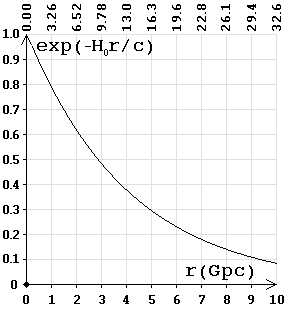Frequency (energy) dependency on the distance. On top of the chart is the time (billion years).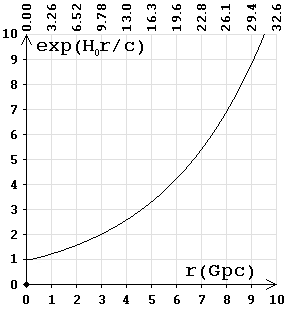Wavelength dependency on the distance. On top of the chart is the time (billion years).

The graph shows that over 9.3 billion years (2.9 gigaparsecs) the frequency is reduced by half. The frequency of the photon is directly proportional to the energy; respectively, E(t) = E0e-H0t - is the formula for photon attenuation, where the Hubble constant represents the parameter of the attenuation of electromagnetic oscillations. This provides total agreement with the exponential law of damped oscillations.

«DAMPED OSCILLATIONS, the natural oscillations, the amplitude A of which decreases with time t according to the law of the exponential A(t) = A0e-at (a - parameter of the attenuation) due to the energy dissipation because of the viscous friction forces for damping mechanical vibrations and the ohmic resistance for electromagnetic damped oscillations.»

The study of the work of Nobel Prize winners in 2011 on the distant type Ia supernovae, attested that the observed redshift of the frequency for both the near and distant galaxies coincides with v(t) = v0e-H0t (formula of dependency of the photon frequency on time). The expression reflects an exponential law of damped oscillations, where H0 - parameter of the attenuation, which is the amount by which the frequency is reduced in one period of oscillation. That is, if the oscillation period t is t = 1/v0, then, regardless of the frequency it is always v(t) = v0 - H0. For example, for distant galaxies at 3.3 Gpc (t = 10.76 billion years = 3.43·1017 seconds), the observed cosmological redshift would be

z = (v0 - v(t)) / v(t) = v0/v(t) - 1 = 1/e-H0t - 1 = eH0t - 1 = 1.3,

rather than z = 2, as assumed in the theory of Universe's expansion, hence the brightness of the supernova is lower.

«With displacements z = 2 ... speed v = 0.8 c ... the distance by the redshift - is r = 3.3 Gpc (the Doppler effect)»
http://ru.wikipedia.org/wiki/Космологическое_красное_смещение (In Russian)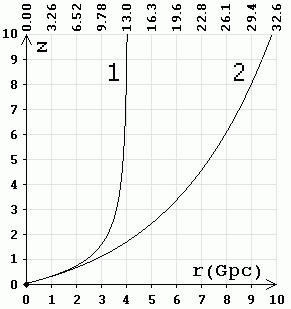1 - according to the theory of the Universe expansion (the Doppler effect). 2 - according to the Hubble quantum law (by the method of "standard candles"). On top of the chart is the time (billion years).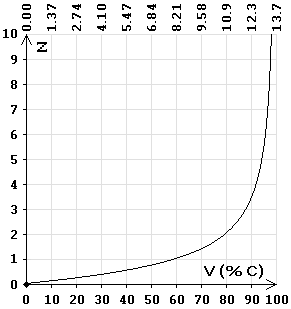Speed dependence on the redshift by the Doppler effect (as percentage of the light speed). On top of the chart is the time (billion years).

«In the works of the Nobel Prize winners in 2011 it was discovered that the far away galaxies, the distance to which was calculated by the Hubble's law, the type Ia supernova's have a brightness lower than the one they were supposed to have. In other words, the distance to these galaxies calculated by the method of the "standard candles" turns out to be larger than the distance calculated on the basis of the previously set value of the Hubble parameter. It was concluded that the Universe is not just expanding, but that it is expanding with acceleration!»
http://hepd.pnpi.spb.ru/ioc/ioc/line%209-10-2011/n5.htm (In Russian)

«Thus, by studying distant from Earth supernovae's, the winners found that those at least a quarter fainter than it had been predicted by the theory, that means the stars are farther than determined from the calculations.»
http://lebed.com/2011/art5913.htm (In Russian)

So studying distant supernovae's, the winners have found that the distance calculated by the Doppler Effect does not correspond with the actual distance to the stars. As of today Ia supernovae's are observed up to z = 2. At z = 2, the distance calculated by the Doppler effect, is 10.8 billion light-years (3.3 Gpc), and by the method of "standard candles" 14.8 billion light-years (4.5 Gpc) redshift increases exponentially z = eH0t - 1. Hence usually a wrong conclusion is made that the Universe is expanding exponentially.

«... the scale factor of the Universe behaves asymptotically like this: a(t) ~ eH0t - the Universe will expand exponentially, and this has not really been expected earlier. That is, it is the accelerated expansion of the Universe, but before that, according to the standard theory, it appeared that the Universe should expand with the slowdown»
Lecture A.D.Linde. http://elementy.ru/lib/430484 (In Russian)

According to the method of "standard candles" the following is established: z + 1 = a0/a(t) = eH0t and z = eH0t - 1, that is the astronomical observations attest that the redshift is subject to exponential law of damped oscillations.

«... a(t) ~ eH0t ... z + 1 = a0/a(t), where a(t) - cosmological scale factor at time t, and a0 - its value at the moment.»
http://www1.jinr.ru/Pepan/2012-v43/v-43-3/01_dol.pdf (In Russian)

During the studies of distant galaxies it was discovered that cosmological redshift occurs according to the law of the exponentially damped oscillations, in accordance with the Hubble's quantum law, not with the Doppler effect, then - for the sake of the salvation of the Big Bang theory and against all laws of physics - a 'miraculous' explanation was invented: the accelerated expansion of the Universe due to dark energy (force). But in this case, the speed of the young galaxies must be less than the speed of the old ones, such as our galaxy, and, therefore, our time should slow down more. Because of this, the observed (by us) duration of the outbreaks of the distant supernovae's should decrease, and not increase.

«This way the galaxies, spectrum of which had the observed double increase of the wavelength of light, had their supernova explosions extended also by two times: from two weeks to four weeks.»
http://www.ritz-btr.narod.ru/compress.html (In Russian)

For each shift, red or purple (due to the Doppler effect, or under the influence of gravity, etc.), always changes not only the wavelength, but also the duration of the wave packets. This happens because the number of periods of oscillation does not change and accordingly, the duration of the wave packets (bursts) varies in direct proportion to the wavelength λ/λ0 = 1 + z.

It is foolish to talk about the expansion with acceleration, if - no matter where we look - according to the Doppler effect, the young (distant) galaxies are moving faster than the old ones, that is to say that over time the speed becomes less - braking is 22 km/s in a million years. At the same time - in some magical way - the young galaxies right after the Big Bang happened to be on the outskirts of the Universe. It turns out that in the first seconds after the explosion, all matter was on the surface of a sphere with a radius of 13.7 billion light-years and was moving away from the center with great speed.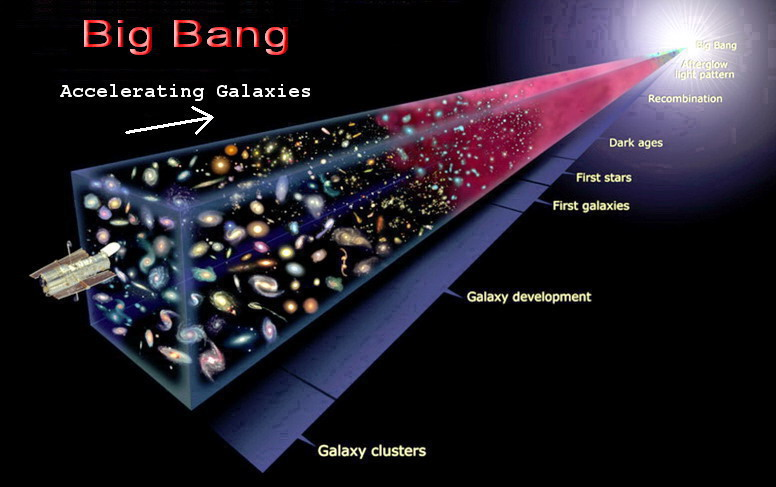According to the theory of Universe expansion, the younger the galaxy the faster it is moving away from us. That is, the acceleration of the galaxies occurs in the direction of the very first seconds after the Big Bang, and this acceleration is observed in any direction from us, indicating the absence of any logic in the theory. It turns out that the explosion is coming from the point of the Big Bang, and conversely, the acceleration occurs on any side of us. The proponents of this theory explain this absurdity by the fact that at the moment of the Big Bang all laws of physics and logic can be broken, and therefore there is "complete freedom of choice".

«At the point of the Big Bang and other singularities all the laws are violated therefore God keeps complete freedom of choice of what was happening in singularities and how the beginning of the Universe looked like.»
Stephen William Hawking.

Also, the statement that the cosmic microwave background radiation is associated with the Big Bang, contradicts logic, as in this case it would have been observed on one side; on the side of the explosion, the radiation source. For example, if somewhere a star explodes, one point would be seen, the one where the explosion occurred. Radiation always comes from the point of radiation. It is applicable to all sources, even those that are more than 13 billion years old; they are also seen as points.

Due to the fact that on the basis of the theory of Universe expansion, the distance calculated using the Doppler effect is wrong, the distant galaxies appear to be small (compact), and type Ia supernovae have lower brightness. For example, galaxy UDFj-39546284, where z = 11.9, according to Hubble's quantum law, the distance is t = ln(z + 1)/H0 = 1.06·1018 sec = 33.8 billion light-years (10.4 Gpc), not 13.4 (4.1 Gpc), as considered in the expansion theory. That is why it seems compact.

«UDFj-39546284 is a compact galaxy, consisting of blue stars that have existed 13.4 billion years ago, that is, approximately 380 million years after the Big Bang. ... The galaxy has a redshift of z = 11.9.»
http://ru.wikipedia.org/wiki/UDFj-39546284 (In Russian)

According to the Big Bang theory, at z = 11.9 using the Doppler effect, the speed of the galaxy is close to the speed of light and time passes very slowly, all processes are slowed down z + 1 = 12.9 times. Accordingly, it turns out that we have aged 380 million years, and galaxy has aged 29.5 million years after the Big Bang. But the formation of galaxies requires about a billion years, and in such a short time the galaxy cannot be formed, and if we consider that by the Doppler effect the distance to distant galaxies is calculated incorrectly, time becomes negative.

«1 billion years - formation of the first galaxies.»
http://www.modcos.com/articles.php?id=105 (In Russian)

Waves are oscillations of a medium, as otherwise diffraction and interference would not been observed. During the oscillations there cannot be one hundred percent energy conversion, so the law of attenuation is effective for all waves, due to dissipation by conversion of one form of energy into another and back. No need to create myths, explaining the cosmological redshift by the intervention of dark energy, "wedging the galaxies off", since the usual formula of damped electromagnetic oscillations, taking into account the quantum principles, allows accurate calculation of the redshift for any distance.

«The chek-up showed that the data almost perfectly coincides with the model of "tired light" and strongly disagrees with Friedmann's model.»
http://www.vokrugsveta.ru/vs/article/6797/ (In Russian)
«Finally, after taking pictures of very distant objects (z of order 4) with the space telescope "Hubble", when it was expected to see the separate objects of the early Universe, close to the beginning of evolution, it became clear, that the complex structures (galaxies) are seen again. It is clear that it would take tremendous time for the formation of its structure, compatible with the age of the Universe calculated for the Big Bang model.»
http://bourabai.narod.ru/shtyrkov/evolution.htm (In Russian)
«... there is no significant evolution of the metallicity of the GRB host galaxies in the range 0 < z < 6.»
A report in the SAI 05.04.13. http://sed.sao.ru/~vo/cosmo_school/presentations/Sokolov_paper.pdf (In Russian)

One of the main tasks of the space telescope "Hubble" was to see the border of the Universe, but it has not been found. Everywhere there is roughly the same pattern of galaxies, which do not differ by age. The Universe is homogeneous, isotropic, there is no evolution of metallicity and no visible signs of the Big Bang.

Distance calculations by the method of "standard candles" showed that the cosmological redshift is in exact accordance with the formulas of Hubble's quantum law. In fact it turns out that the Nobel Prize in 2011 has been awarded for the confirmation of the Hubble's quantum law.

Hubble's law, as presented in quantum form, allows exact calculation of the cosmological redshift for galaxies at any distance. For example, the distance to galaxies, as calculated on the basis of the contemporary method of "standard candles" (Nobel Prize 2011) mismatches the one calculated on the basis of the Doppler effect (the Big Bang theory), while it is fully consistent with Hubble's quantum law.

«It may seem that the vacuum fluctuations is an abstraction occuring in the sick brain of the physicist, but it is not like that. The observable manifestations of the vacuum fluctuation can be experimentally detected in the microcosm.»
http://scorcher.ru/art/theory/vacuum/vacuum.php (in Russian)

In quantum physics some of the phenomena may at first glance seem absurd, for example wave-particle duality. Same is true for the virtual particles; in spite of the apparent "nonsense" the quantum vacuum fluctuations do actually manifest themselves in the Casimir effect, the vacuum polarization, the spontaneous photon emission and the electrons' "trembling" on the atomic orbits. Quantum fluctuations affect all elementary particles, and, respectively, travelling through the fluctuating vacuum spaceships "shake" as if traveling on the uneven road, which results in their braking. On this moment the existence of the quantum vacuum fluctuations is an experimentally proved fact. That is to say that the vacuum is not just an empty space, but the "sea" of the virtual particles, therefore we should take into account their influence not only on the microparticles, but also on the cosmic objects. Concluding, it can be said that from the position of the quantum physics, there is nothing phenomenal in the fact of such vacuum braking. Abroad this field is being researched.

«Physicists predicted vacuum resistance.»
http://www.membrana.ru/particle/15726 (in Russian)
«Inhibition of the heavenly bodies particles of dark matter»
Department of Particle Physics and Cosmology, Physics Department of MSU. http://ppc.inr.ac.ru/defence_2kurs.php (in Russian)

On the contrary, it would have been strange, if moving body would not have been affected by the constant influence of the virtual particles.

«Real particles constantly undergo the influence of the virtual ones, and, therefore, unable to stay still. They behave as Brownian particles. As the result of the influence of the virtual particles on the real particles, the trajectories of the movement of the last ones are indistinct (is seen as lamb shift of the electron's energy levels in atom). ... Virtual pairs particle-anti-particle constantly appear and disappear, this phenomenon is seen as vacuum fluctuations.»

At the motion of bodies such influence from the virtual particles creates a pressure difference, which leads to inhibition. It should be noted that, the certain inhibition in the vacuum arises relatively to the microwave background radiation, since the electromagnetic waves also create pressure. And even just starlight cause inhibition, because the higher speed is, the greater the number of stars will be seen in front than behind.

A few years ago (2008), when nobody was doing research on the friction in the vacuum (at least there were no publications) and this work was perhaps the only one, the very fact of the possibility of the friction in the vacuum was considered not acceptable and met with hostility by the adherents of the classical concept of the vacuum as an empty space. But when other scientists began to engage in similar researches and some publications appeared on the friction (inhibition) in the vacuum, views on this subject began to change. And in 2011 direct experimental evidence was obtained, that is it was experimentally confirmed that a moving body "pushes" forward virtual particles (dynamical Casimir effect) and, therefore, looses its speed.

«First of all, we would like to thank you for such a courageous and pioneering article "Braking of the space crafts caused by the vacuum fluctuations". The reading of this article led to the series of discussions in our organization. To the essence of the subject we can answer the following. The main result of your work, namely - the formula for calculating the energy dissipation during the motion of various objects in the physical vacuum - does not simply attempt to explain some of the observed experimental deviations from the existing theoretical models of similar motion. It claims to replace the existing paradigm of the scientific picture of the world! We are talking here about the change of the speed of the bodies relative to the physical vacuum. And this problem has already engaged thousands of researchers during two centuries!»
Russian Federal Space Agency (ROSCOSMOS)

Braking of the space crafts caused by the vacuum fluctuations (Word)

Braking of the space crafts caused by the vacuum fluctuations (in Russian)
Трение космических аппаратов о флуктуации вакуума

Hubble's quantum law (in English)       Hubble's quantum law (in Russian)
Published in the «Engineering Physics» (No. 3, 2014)